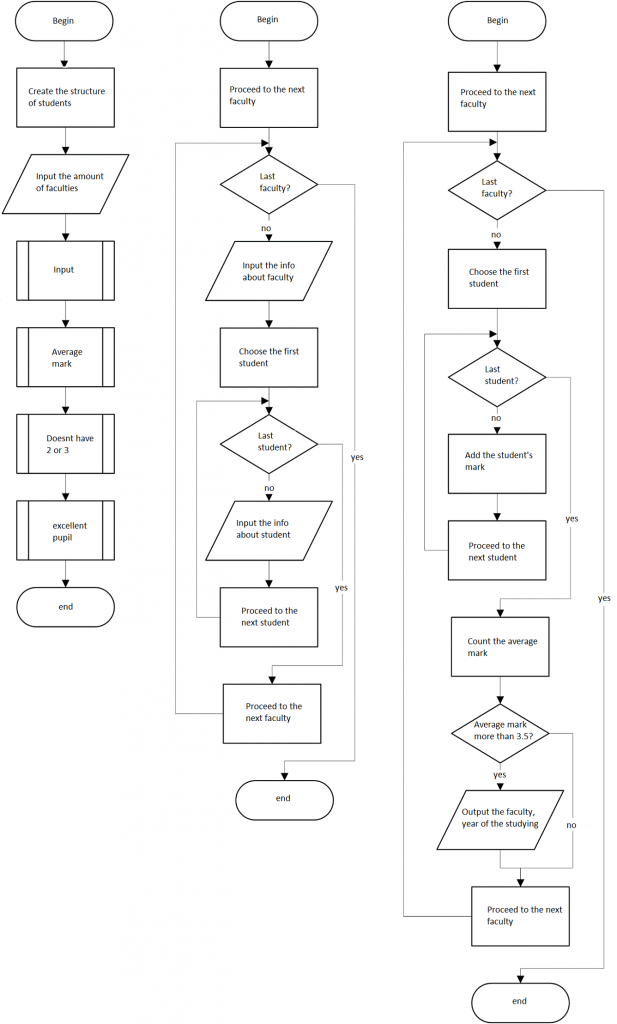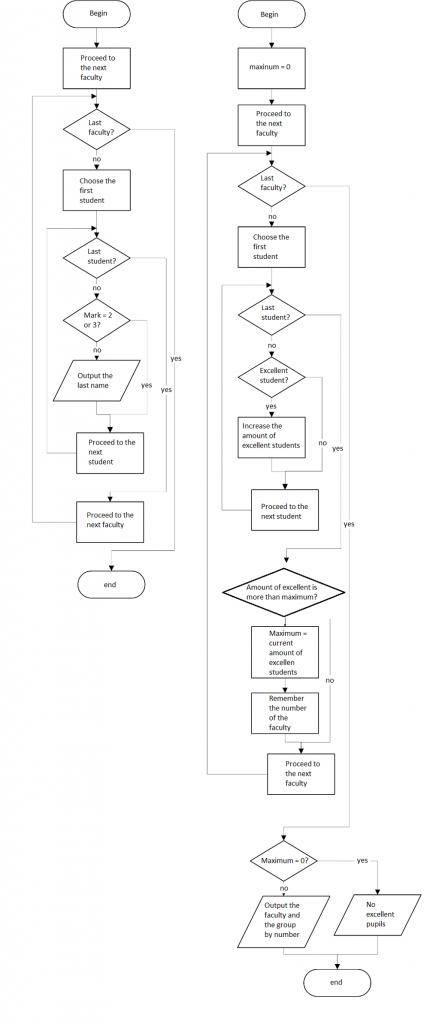# Assignment Examples Free: Structures and Structure Arrays

Task: Create an array of structures. Each structure consists of the following elements: faculty, course, group, names of students, test scores. Implement requests, determining: 1) Faculty and course where the average score of at least 3.5; 2) the names of students who have twos and threes; 3) group and the faculty, with high amount excellent pupils.
Block schemes of the solution:С++ implementation:

``` #include <iostream>
#include <conio.h>
#include <stdio.h>
using namespace std;
const int students_num = 4;//number of students in faculty

struct surnames//student
{
char surname;//last name
bool without23;//no 2 or 3
bool excelStudent;
};

struct pupil//faculty
{
char faculty;//name of faculty
int course;
char group;
float average;//average mark
int exStudentNumber;//amount of excellent pupils
surnames surname[students_num];//students
};
void input_average(pupil *arr, int num)//input date and calculate average and amount of excellent students
{
int sumAverage = 0, excelStudentSum;
for (int i = 0; i < num; i++)
{
sumAverage = 0;
excelStudentSum = 0;
cout << "faculty: "; cin >> arr[i].faculty;
cout << "course: "; cin >> arr[i].course;
cout << "group: "; cin >> arr[i].group;
for (int k = 0; k < students_num; k++)
{
cout << k + 1 << " student:" << endl;
cout << " surname: "; cin >> arr[i].surname[k].surname;
sumAverage += arr[i].surname[k].grade;//sum of the marks
arr[i].surname[k].without23 = 0;
else arr[i].surname[k].without23 = 1;
arr[i].surname[k].excelStudent = 1;
else
arr[i].surname[k].excelStudent = 0;
excelStudentSum += arr[i].surname[k].excelStudent;//amount of excellent students
}
arr[i].average = (float)sumAverage / students_num;//calculate average
arr[i].exStudentNumber = excelStudentSum;
cout << endl << endl;
}
}
void output_students(pupil *arr, int num)//output students without 2,3
{
bool flag = 0;
cout << "students without 2 & 3:" << endl;
for (int i = 0; i < num; i++)
for (int k = 0; k < students_num; k++)
if (arr[i].surname[k].without23)
{
cout << '\t' << arr[i].surname[k].surname << endl;
flag = 1;
}
if (!flag)
cout << " " << "empty" << endl; } void output_facultets(pupil *arr, int num)//output facultets with average>3.5
{
bool flag = 0;
cout << "facultets with average grades >= 3.5:" << endl;
for (int i = 0; i < num; i++) if (arr[i].average >= 3.5)//average >3.5?
{
cout << " " << "faculty: " << arr[i].faculty << endl << " " << "course: " << arr[i].course << endl;
flag = 1;
}
if (!flag)
cout << " " << "empty" << endl;
}
void output_vidminnuk(pupil *arr, int num)//output faculty with max excellent students
{
int max = 0;
int index_max = 0;
cout << "max excellent students:" << endl;
for (int i = 0; i < num; i++) if (arr[i].exStudentNumber > max)
{
max = arr[i].exStudentNumber;
index_max = i;
}
if (max != 0)
cout << " faculty: " << arr[index_max].faculty << endl << " group: " << arr[index_max].group << endl;
else
cout << " No excellent students" << endl;
}

void main()
{
int num;
cout << "enter number of facultets: "; cin >> num;
pupil *arr = new pupil[num];
input_average(arr, num);
output_facultets(arr, num);
output_students(arr, num);
output_vidminnuk(arr, num);
system("pause");
}

```

We hope you like our assignments examples, free samples are presented only for viewing. For example, you can use our extended Euclidean algorithm example to deal with your own homework. Hundreds of students have difficulties with their assignments – that’s why we offer professional help from AssignmentShark.com. It is always best to get professional help than struggling on your own not knowing what to do. Every order on our site is done with individual approach by experts in different fields of study. We have reasonable prices, so that any student can afford using our service. Get our help and forget about your assignment problems!